# Image Approximation with Fourier and Wavelets¶

Important: Please read the installation page for details about how to install the toolboxes. $\newcommand{\dotp}{\langle #1, #2 \rangle}$ $\newcommand{\enscond}{\lbrace #1, #2 \rbrace}$ $\newcommand{\pd}{ \frac{ \partial #1}{\partial #2} }$ $\newcommand{\umin}{\underset{#1}{\min}\;}$ $\newcommand{\umax}{\underset{#1}{\max}\;}$ $\newcommand{\umin}{\underset{#1}{\min}\;}$ $\newcommand{\uargmin}{\underset{#1}{argmin}\;}$ $\newcommand{\norm}{\|#1\|}$ $\newcommand{\abs}{\left|#1\right|}$ $\newcommand{\choice}{ \left\{ \begin{array}{l} #1 \end{array} \right. }$ $\newcommand{\pa}{\left(#1\right)}$ $\newcommand{\diag}{{diag}\left( #1 \right)}$ $\newcommand{\qandq}{\quad\text{and}\quad}$ $\newcommand{\qwhereq}{\quad\text{where}\quad}$ $\newcommand{\qifq}{ \quad \text{if} \quad }$ $\newcommand{\qarrq}{ \quad \Longrightarrow \quad }$ $\newcommand{\ZZ}{\mathbb{Z}}$ $\newcommand{\CC}{\mathbb{C}}$ $\newcommand{\RR}{\mathbb{R}}$ $\newcommand{\EE}{\mathbb{E}}$ $\newcommand{\Zz}{\mathcal{Z}}$ $\newcommand{\Ww}{\mathcal{W}}$ $\newcommand{\Vv}{\mathcal{V}}$ $\newcommand{\Nn}{\mathcal{N}}$ $\newcommand{\NN}{\mathcal{N}}$ $\newcommand{\Hh}{\mathcal{H}}$ $\newcommand{\Bb}{\mathcal{B}}$ $\newcommand{\Ee}{\mathcal{E}}$ $\newcommand{\Cc}{\mathcal{C}}$ $\newcommand{\Gg}{\mathcal{G}}$ $\newcommand{\Ss}{\mathcal{S}}$ $\newcommand{\Pp}{\mathcal{P}}$ $\newcommand{\Ff}{\mathcal{F}}$ $\newcommand{\Xx}{\mathcal{X}}$ $\newcommand{\Mm}{\mathcal{M}}$ $\newcommand{\Ii}{\mathcal{I}}$ $\newcommand{\Dd}{\mathcal{D}}$ $\newcommand{\Ll}{\mathcal{L}}$ $\newcommand{\Tt}{\mathcal{T}}$ $\newcommand{\si}{\sigma}$ $\newcommand{\al}{\alpha}$ $\newcommand{\la}{\lambda}$ $\newcommand{\ga}{\gamma}$ $\newcommand{\Ga}{\Gamma}$ $\newcommand{\La}{\Lambda}$ $\newcommand{\si}{\sigma}$ $\newcommand{\Si}{\Sigma}$ $\newcommand{\be}{\beta}$ $\newcommand{\de}{\delta}$ $\newcommand{\De}{\Delta}$ $\newcommand{\phi}{\varphi}$ $\newcommand{\th}{\theta}$ $\newcommand{\om}{\omega}$ $\newcommand{\Om}{\Omega}$

This numerical tour overviews the use of Fourier and wavelets for image approximation.

In :
from __future__ import division

import numpy as np
import scipy as scp
import pylab as pyl
import matplotlib.pyplot as plt

from nt_toolbox.general import *
from nt_toolbox.signal import *

import warnings
warnings.filterwarnings('ignore')

%matplotlib inline


Note: to measure the error of an image $f$ with its approximation $f_M$, we use the SNR measure, defined as

$$\text{SNR}(f,f_M) = -20\log_{10} \pa{ \frac{ \norm{f-f_M} }{ \norm{f} } },$$

which is a quantity expressed in decibels (dB). The higer the SNR, the better the quality.

First we load an image $f \in \RR^N$ of $N = N_0 \times N_0$ pixels.

In :
n0 = 512


Display the original image.

In :
plt.figure(figsize = (5,5))
imageplot(f, 'Image f')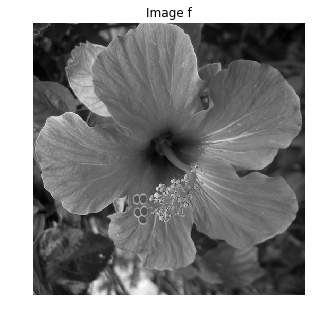Display a zoom in the middle.

In :
plt.figure(figsize = (5,5))
imageplot(f[n0//2 - 32:n0//2 + 32,n0//2 - 32:n0//2 + 32], 'Zoom')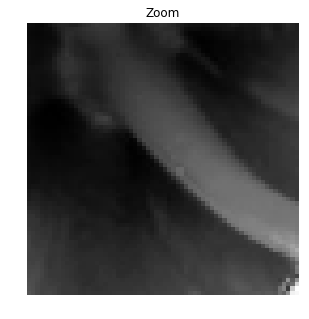An image is a 2D array, it can be modified as a matrix.

In :
plt.figure(figsize = (8,8))
imageplot(-f, '-f', [1, 2, 1])
imageplot(f[::-1,], 'Flipped', [1, 2, 2])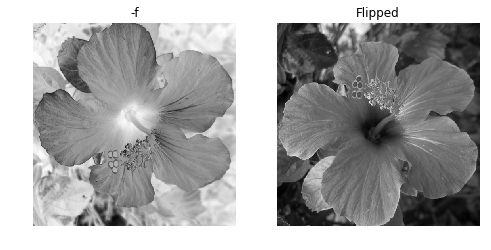Blurring is achieved by computing a convolution $f \star h$ with a kernel $h$.

Compute the low pass kernel.

In :
k = 9; #size of the kernel
h = np.ones([k,k])
h = h/np.sum(h) #normalize


Compute the convolution $f \star h$.

In :
from scipy import signal
fh = signal.convolve2d(f, h, boundary = "symm")


Display.

In :
plt.figure(figsize = (5,5))
imageplot(fh, 'Blurred image')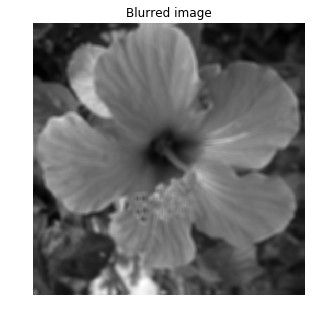## Fourier Transform¶

The Fourier orthonormal basis is defined as $$\psi_m(k) = \frac{1}{\sqrt{N}}e^{\frac{2i\pi}{N_0} \dotp{m}{k} }$$ where $0 \leq k_1,k_2 < N_0$ are position indexes, and $0 \leq m_1,m_2 < N_0$ are frequency indexes.

The Fourier transform $\hat f$ is the projection of the image on this Fourier basis

$$\hat f(m) = \dotp{f}{\psi_m}.$$

The Fourier transform is computed in $O(N \log(N))$ operation using the FFT algorithm (Fast Fourier Transform). Note the normalization by $\sqrt{N}=N_0$ to make the transform orthonormal.

In :
F = pyl.fft2(f)/n0


We check this conservation of the energy.

In :
from pylab import linalg

print("Energy of Image:   %f" %linalg.norm(f))
print("Energy of Fourier: %f" %linalg.norm(F))

Energy of Image:   205.747421
Energy of Fourier: 205.747421


Compute the logarithm of the Fourier magnitude $\log\left(\abs{\hat f(m)} + \epsilon\right)$, for some small $\epsilon$.

In :
L = pyl.fftshift(np.log(abs(F) + 1e-1))


Display. Note that we use the function fftshift to put the 0 low frequency in the middle.

In :
plt.figure(figsize = (5,5))
imageplot(L, 'Log(Fourier transform)')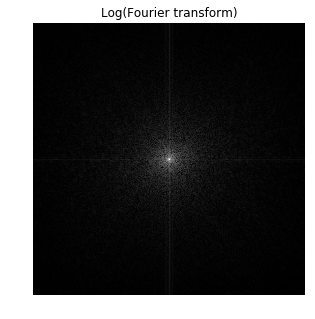## Linear Fourier Approximation¶

An approximation is obtained by retaining a certain set of index $I_M$

$$f_M = \sum_{ m \in I_M } \dotp{f}{\psi_m} \psi_m.$$

Linear approximation is obtained by retaining a fixed set $I_M$ of $M = \abs{I_M}$ coefficients. The important point is that $I_M$ does not depend on the image $f$ to be approximated.

For the Fourier transform, a low pass linear approximation is obtained by keeping only the frequencies within a square.

$$I_M = \enscond{m=(m_1,m_2)}{ -q/2 \leq m_1,m_2 < q/2 }$$

where $q = \sqrt{M}$.

This can be achieved by computing the Fourier transform, setting to zero the $N-M$ coefficients outside the square $I_M$ and then inverting the Fourier transform.

Number $M$ of kept coefficients.

In :
M = n0**2//64


Exercise 1

Perform the linear Fourier approximation with $M$ coefficients. Store the result in the variable $f_M$.

In :
run -i nt_solutions/introduction_4_fourier_wavelets/exo1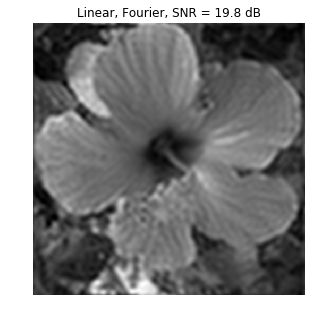In :
## Insert your code here.


Compare two 1D profile (lines of the image). This shows the strong ringing artifact of the linea approximation.

In :
plt.figure(figsize=(7,6))

plt.subplot(2, 1, 1)
plt.plot(f[: , n0//2])
plt.xlim(0,n0)
plt.title('f')

plt.subplot(2, 1, 2)
plt.plot(fM[: , n0//2])
plt.xlim(0,n0)
plt.title('f_M')

plt.show()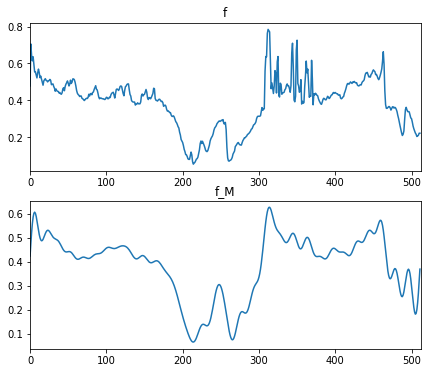## Non-linear Fourier Approximation¶

Non-linear approximation is obtained by keeping the $M$ largest coefficients. This is equivalently computed using a thresholding of the coefficients $$I_M = \enscond{m}{ \abs{\dotp{f}{\psi_m}}>T }.$$

Set a threshold $T>0$.

In :
T = .2


Compute the Fourier transform.

In :
F = pyl.fft2(f)/n0


Do the hard thresholding.

In :
FT = np.multiply(F,(abs(F) > T))


Display. Note that we use the function fftshift to put the 0 low frequency in the middle.

In :
L = pyl.fftshift(np.log(abs(FT) + 1e-1))
plt.figure(figsize = (5,5))
imageplot(L, 'thresholded Log(Fourier transform)')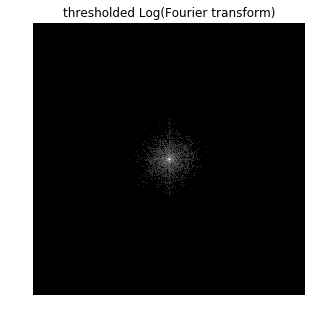Inverse Fourier transform to obtain $f_M$.

In :
fM = np.real(pyl.ifft2(FT)*n0)


Display.

In :
plt.figure(figsize = (5,5))
imageplot(clamp(fM), "Linear, Fourier, SNR = %.1f dB" %snr(f, fM))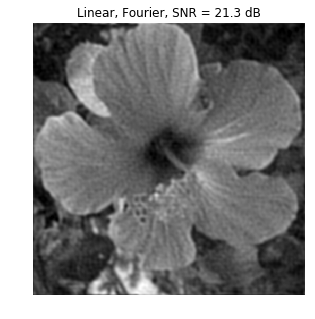Given a $T$, the number of coefficients is obtained by counting the non-thresholded coefficients $\abs{I_M}$.

In :
m = np.sum(FT != 0)
print('M/N = 1/%d'  %(n0**2/m))

M/N = 1/53


Exercise 2

Compute the value of the threshold $T$ so that the number of coefficients is $M$. Display the corresponding approximation $f_M$.

In :
run -i nt_solutions/introduction_4_fourier_wavelets/exo2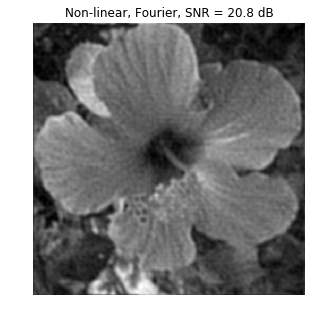In :
## Insert your code here.


## Wavelet Transform¶

A wavelet basis $\Bb = \{ \psi_m \}_m$ is obtained over the continuous domain by translating and dilating three mother wavelet functions $\{\psi^V,\psi^H,\psi^D\}$.

Each wavelet atom is defined as $$\psi_m(x) = \psi_{j,n}^k(x) = \frac{1}{2^j}\psi^k\pa{ \frac{x-2^j n}{2^j} }$$

The scale (size of the support) is $2^j$ and the position is $2^j(n_1,n_2)$. The index is $m=(k,j,n)$ for $\{ j \leq 0 \}$.

The wavelet transform computes all the inner products $\{ \dotp{f}{\psi_{j,n}^k} \}_{k,j,n}$.

Set the minimum scale for the transform to be 0.

In :
Jmin = 0


Perform the wavelet transform, $f_w$ stores all the wavelet coefficients.

In :
from nt_toolbox.perform_wavelet_transf import *

fw = perform_wavelet_transf(f, Jmin, + 1)


Display the transformed coefficients.

In :
plt.figure(figsize=(10,10))

plot_wavelet(fw)
plt.title('Wavelet coefficients')

plt.show()